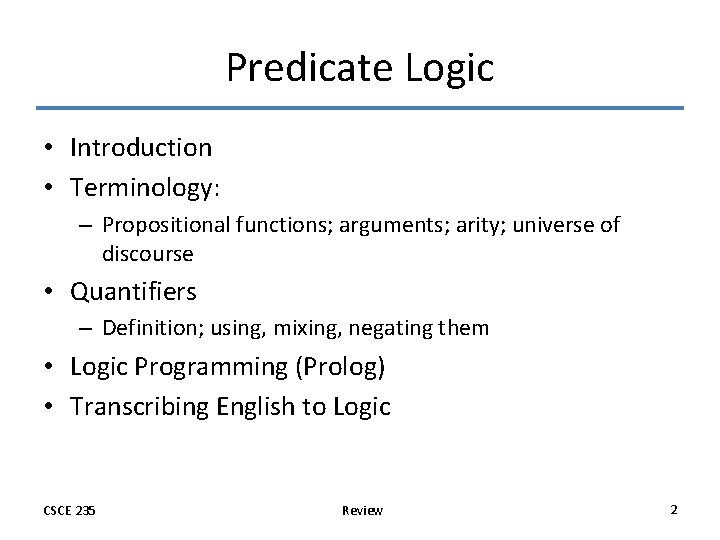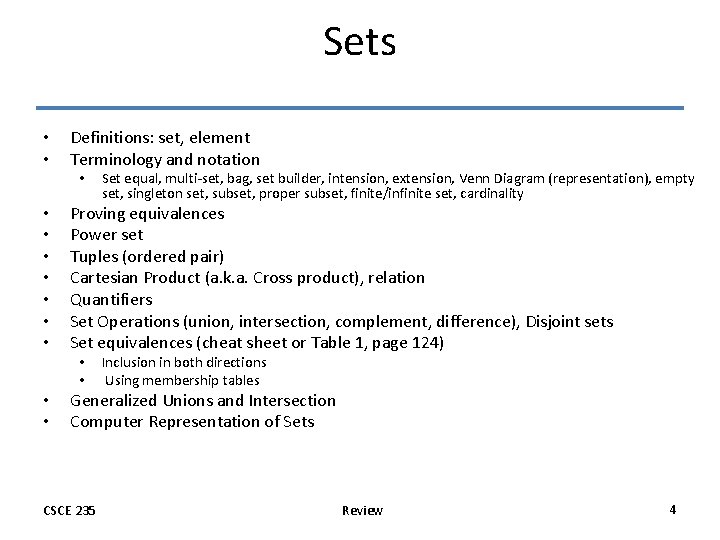# Propositional Logic Defining Propositional Logic Propositions Connectives Truth

• Slides: 5Propositional Logic • Defining Propositional Logic – Propositions – Connectives – Truth tables • Precedence of Logical Operators • Usefulness of Logic – Bitwise operations – Logic in Theoretical Computer Science (SAT) – Logic in Programming • Logical Equivalences – Terminology – Truth tables – Equivalence rules CSCE 235 Review 1Predicate Logic • Introduction • Terminology: – Propositional functions; arguments; arity; universe of discourse • Quantifiers – Definition; using, mixing, negating them • Logic Programming (Prolog) • Transcribing English to Logic CSCE 235 Review 2Proofs • Terminology • Rules of inference: • Modus ponens, addition, simplification, conjunction, modus tollens, contrapositive, hypothetical syllogism, disjunctive syllogism, resolution, • Examples • Fallacies • Proofs with quantifiers • Types of proofs: • Trivial, vacuous, direct, by contrapositive (indirect), by contradiction (indirect), by cases, existence and uniqueness proofs; counter examples • Proof strategies: • Forward reasoning; Backward reasoning; Alerts CSCE 235 Review 3Sets • • Definitions: set, element Terminology and notation • • Proving equivalences Power set Tuples (ordered pair) Cartesian Product (a. k. a. Cross product), relation Quantifiers Set Operations (union, intersection, complement, difference), Disjoint sets Set equivalences (cheat sheet or Table 1, page 124) • • Set equal, multi-set, bag, set builder, intension, extension, Venn Diagram (representation), empty set, singleton set, subset, proper subset, finite/infinite set, cardinality Inclusion in both directions Using membership tables Generalized Unions and Intersection Computer Representation of Sets CSCE 235 Review 4Functions • Definitions & terminology – function, domain, co-domain, image, preimage (antecedent), range, image of a set, strictly increasing, strictly decreasing, monotonic • Properties – One-to-one (injective), onto (surjective), one-to-one correspondence (bijective) – Exercices (5) • Inverse functions (examples) • Operators – Composition, Equality • Important functions – identity, absolute value, floor, ceiling, factorial CSCE 235 Review 5